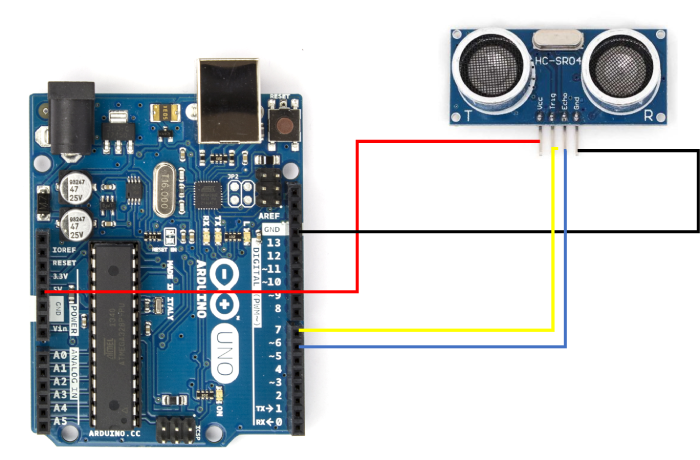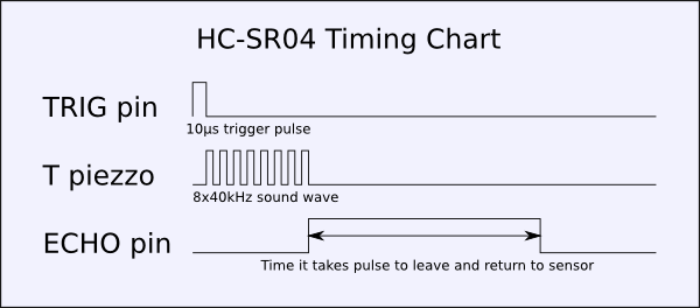# Interfacing an ultrasonic sensor with Arduino

In this tutorial, we will interface the ultrasonic sensor HC-SR04 with the Arduino to get the distance from a surface in centimetres.

## Circuit DiagramAs you can see, you need to connect the Vcc pin of HC-SR04 to 5V, GND to GND, Trig pin to pin 7 of Arduino Uno, and Echo pin to pin 6 of Arduino. You can actually choose any GPIO instead of pins 7 and 6. You just have to make sure that the definitions in the code are proper.

## Working of HC-SR04

The HC-SR04 emits ultrasonic waves at 40,000 Hz. In order to make it emit waves, we need to give a 10 microseconds HIGH pulse at the Trigger pin. The module responds by emitting a sonic burst of 8 pulses. This 8-pulse pattern helps differentiate the pulses emitted by the module from the ambient noise. As soon as the pulses are transmitted, the ECHO pin goes HIGH, and stays HIGH till all the reflected pulses are received. The module times out after 38ms, if all the reflected pulses are not received in this duration.The following timing diagram explains the behavior of the module −

The time for which the Echo pin remains HIGH can help determine the distance of the sensor from the reflecting surface. The speed of sound in air is 340 m/s, or 0.034 cm/ microsecond. If the ECHO pin stays HIGH for, say 100 microseconds, then the distance travelled by the waves is: 100*0.034 = 3.4cm. Therefore, the distance from the surface is 3.4/2 = 1.7 cm (since the waves reflect back from the surface and cover the same distance again)

## Example

The entire code is given below −

#define echoPin 6
#define triggerPin 7

void setup() {
pinMode(triggerPin, OUTPUT);       // Sets the trigPin as an OUTPUT
pinMode(echoPin, INPUT);          // Sets the echoPin as an INPUT
Serial.begin(9600);             // Serial Communication is starting with 9600 of baudrate speed
Serial.println("Ultrasonic Sensor HC-SR04 Test"); // print some text in Serial Monitor

Serial.println("with Arduino UNO");
}
void loop() {
long highPulseDuration;
int calculatedDistanceCm;

//Set the trigPin to low, before setting it to high for the pulse
digitalWrite(triggerPin, LOW);
delayMicroseconds(5);

// Create the 10 seconds pulse on the trig pin
digitalWrite(triggerPin, HIGH);
delayMicroseconds(10);

// Set the pin to low to end the pulse
digitalWrite(triggerPin, LOW);

// Read the duration of the high pulse on the echo pin
highPulseDuration = pulseIn(echoPin, HIGH);

// Calculating the distance
calculatedDistanceCm = highPulseDuration * 0.034 / 2; // Speed of
sound wave divided by 2 (go and back)

// Displays the distance on the Serial Monitor
Serial.print("Calculated Distance: ");
Serial.print(calculatedDistanceCm);
Serial.println(" cm");
}

As you can see, we begin with the definitions of echo and trigger pins.

#define echoPin 6
#define triggerPin 7

Within the setup, we set the echoPin as input, and trigger pin as output, and initialize Serial.

void setup() {
pinMode(triggerPin, OUTPUT); // Sets the trigPin as an OUTPUT
pinMode(echoPin, INPUT); // Sets the echoPin as an INPUT
Serial.begin(9600); // Serial Communication is starting with 9600 of baudrate speed
Serial.println("Ultrasonic Sensor HC-SR04 Test"); // print some text in Serial Monitor
Serial.println("with Arduino UNO");
}

Within the loop, we do the following −

• Define the variables for measuring the duration of the HIGH pulse

• Clear the trigger pin (by setting it to LOW), then set it to HIGH for 10 microseconds, and then again set it to LOW. This produces a 10 microseconds HIGH pulse from the Trigger pin.

• Next, we record the duration of the HIGH pulse on the echo pin, using the pulseIn function. You can read more about the pulseIn function here.

• From the duration of the ECHO pin pulse, we calculate the distance from the reflecting surface, and print it in cm.

void loop() {
long highPulseDuration;
int calculatedDistanceCm;

//Set the trigPin to low, before setting it to high for the pulse
digitalWrite(triggerPin, LOW);
delayMicroseconds(5);

// Create the 10 seconds pulse on the trig pin
digitalWrite(triggerPin, HIGH);
delayMicroseconds(10);

// Set the pin to low to end the pulse
digitalWrite(triggerPin, LOW);

// Read the duration of the high pulse on the echo pin
highPulseDuration = pulseIn(echoPin, HIGH);

// Calculating the distance
calculatedDistanceCm = highPulseDuration * 0.034 / 2; // Speed of
sound wave divided by 2 (go and back)

// Displays the distance on the Serial Monitor
Serial.print("Calculated Distance: ");
Serial.print(calculatedDistanceCm);
Serial.println(" cm");
}